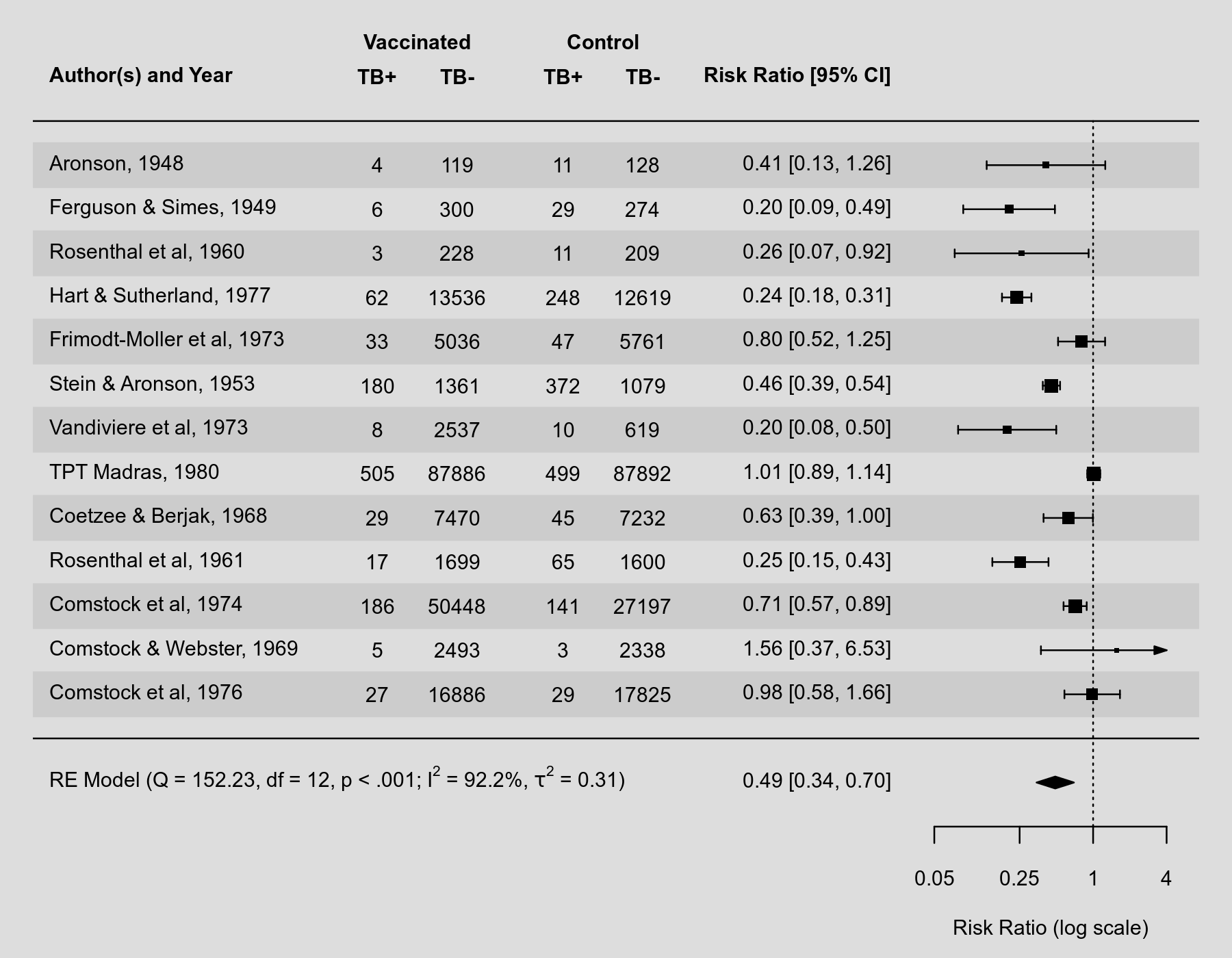#The metafor Package

A Meta-Analysis Package for R

### Site Tools

plots:forest_plot_with_textpos

## Forest Plot with Adjusted Text Position

### Description

By default, the forest() function places the annotations (giving the study-specific estimates and corresponding confidence interval bounds) to the right of the actual forest plot. See here for an example. Sometimes, we might prefer to show those annotations in a different position. This can be done with the textpos argument, which takes as input a vector of length 2 to specify the placement of the study labels and the annotations. The plot below illustrates the use of this argument.

### Plot### Code

library(metafor)

### copy BCG vaccine meta-analysis data into 'dat'
dat <- dat.bcg

### calculate log risk ratios and corresponding sampling variances (and use
### the 'slab' argument to store study labels as part of the data frame)
dat <- escalc(measure="RR", ai=tpos, bi=tneg, ci=cpos, di=cneg, data=dat,
slab=paste(author, year, sep=", "))

### fit random-effects model
res <- rma(yi, vi, data=dat)

### forest plot with extra annotations
forest(res, atransf=exp, at=log(c(0.05, 0.25, 1, 4)), xlim=c(-20,2),
ilab=cbind(tpos, tneg, cpos, cneg), ilab.xpos=c(-13.5,-12,-10,-8.5),
text(c(-13.5,-12,-10,-8.5), res$k+2, c("TB+", "TB-", "TB+", "TB-")) text(c(-12.75,-9.25), res$k+3, c("Vaccinated", "Control"))
"RE Model (Q = ", .(fmtx(res$QE, digits=2)), ", df = ", .(res$k - res$p), ", ", .(fmtp(res$QEp, digits=3, pname="p", add0=TRUE, sep=TRUE, equal=TRUE)), "; ",
I^2, " = ", .(fmtx(res\$I2, digits=1)), "%)")))